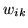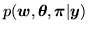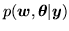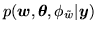Next: Control parameter, Up: Appendix Previous: Gamma Distribution

## MCMC

The three models we want to infer upon are described in section 4. As described in section 4 we infer the marginal posterior distribution for the weights,, by sampling from the full joint posterior distribution, i.e.:for model 1 (equation 9),for model 2 (equation 14) orfor model 3 (equation 18). To sample these full joint posterior distributions we use Markov Chain Monte Carlo (MCMC) techniques. See Gilks et al. (1996) or Gamerman (1997) for an introduction to MCMC sampling. A sample from the joint posterior distributions can be obtained by cycling through and sampling a subset of the parameters at a time. We now describe how we sample from each subset of parameters.

Subsections